# Imaginary numbers

Find two imaginary numbers whose sum is a real number. How are the two imaginary numbers related?
What is its sum?

Correct result:

Sum =  0

#### Solution:

Answer is a conjugate pair of imaginary numbers (its real parts is zero).
The two imaginary numbers that add up to a real number would be ni and -ni, because ni + (-ni) = ni - ni = 0; where 'n' is any real number, no zero. Thus, the sum of this two imaginary numbers become a real number 0.

$ie... \{ 3i; -3i \} \ \\ \Sigma = 3i + (-3i ) = 0$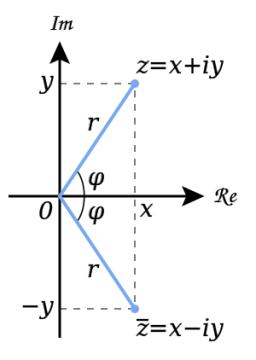Our examples were largely sent or created by pupils and students themselves. Therefore, we would be pleased if you could send us any errors you found, spelling mistakes, or rephasing the example. Thank you!

Leave us a comment of this math problem and its solution (i.e. if it is still somewhat unclear...):

Showing 1 comment:Dr Math
add up a number and its conjugateTips to related online calculators

## Next similar math problems:

• Linear imaginary equationGiven that ? "this is z star" Find the value of the complex number z.
• The modulus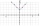Find the modulus of the complex number 2 + 5i
• BearingA plane flew 50 km on a bearing 63°20' and the flew on a bearing 153°20' for 140km. Find the distance between the starting point and the ending point.
• Moivre 2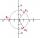Find the cube roots of 125(cos 288° + i sin 288°).
• Is complexAre these numbers 2i, 4i, 2i + 1, 8i, 2i + 3, 4 + 7i, 8i, 8i + 4, 5i, 6i, 3i complex?
• Goniometric form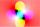Determine goniometric form of a complex number ?.
• Im>0?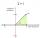Is -10i a positive number?
• Complex number coordinatesWhich coordinates show the location of -2+3i
• ABS CNCalculate the absolute value of complex number -15-29i.
• De Moivre's formula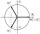There are two distinct complex numbers z such that z3 is equal to 1 and z is not equal 1. Calculate the sum of these two numbers.
• Log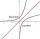Calculate value of expression log |3 +7i +5i2| .
• ReciprocalCalculate reciprocal of z=0.8-1.8i:A domed stadium is in the shape of spherical segment with a base radius of 150 m. The dome must contain a volume of 3500000 m³. Determine the height of the dome at its centre to the nearest tenth of a meter.